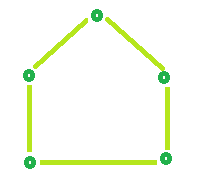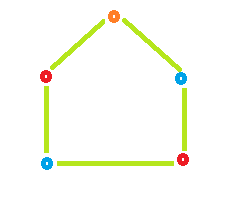# Coloring a Cycle Graph

Cycle:- cycle is a path of edges and vertices wherein a vertex is reachable from itself. or in other words, it is a Closed walk.
Even Cycle:- In which Even number of vertices is present is known as Even Cycle.
Odd Cycle:- In which Odd number of Vertices is present is known as Odd Cycle.

Given the number of vertices in a Cyclic Graph. The task is to determine the Number of colors required to color the graph so that No two Adjacent vertices have the same color.

Approach:

If the no. of vertices is Even then it is Even Cycle and to color such graph we require 2 colors.
If the no. of vertices is Odd then it is Odd Cycle and to color such graph we require 3 colors.

Examples:

```Input : vertices = 3
Output : No. of colors require is: 3

Input : verices = 4
Output : No. of colors require is: 2
```

Example 1: Even Cycle: Number of vertices = 4Color required = 2Example 2: Odd Cycle: Number of vertices = 5Color required = 3## C++

 `// CPP program to find number of colors ` `// required to color a cycle graph ` `#include ` `using` `namespace` `std; ` ` `  `// Function that calculates Color ` `// require to color a graph. ` `int` `Color(``int` `vertices) ` `{ ` `    ``int` `result = 0; ` ` `  `    ``// Check if number of vertices ` `    ``// is odd or even. ` `    ``// If number of vertices is even ` `    ``// then color require is 2 otherwise 3 ` `    ``if` `(vertices % 2 == 0) ` `        ``result = 2; ` `    ``else` `        ``result = 3; ` ` `  `    ``return` `result; ` `} ` ` `  `// Driver code ` `int` `main() ` `{ ` `    ``int` `vertices = 3; ` `    ``cout << ``"No. of colors require is: "` `<< Color(vertices); ` `    ``return` `0; ` `} `

## Java

 `// Java program to find number of colors ` `// required to color a cycle graph ` `import` `java.io.*;  ` ` `  `class` `GFG {  ` `   `  `    ``// Function that calculates Color  ` `    ``// require to color a graph.   ` `    ``static` `int` `Color(``int` `vertices)  ` `    ``{  ` `        ``int` `result = ``0``;  ` `   `  `        ``// Check if number of vertices  ` `        ``// is odd or even.  ` `        ``// If number of vertices is even  ` `        ``// then color require is 2 otherwise 3  ` `        ``if` `(vertices % ``2` `== ``0``)  ` `            ``result = ``2``;  ` `        ``else` `            ``result = ``3``;  ` `       `  `        ``return` `result;  ` `    ``}   ` `       `  `    ``// Driver program to test above function  ` `    ``public` `static` `void` `main (String[] args)  ` `    ``{  ` `        ``int` `vertices = ``3``;  ` `         `  `        ``System.out.println(``"No. of colors require is: "` `+ Color(vertices)); ` `           `  `    ``}  ` `}  ` ` `  `// this code is contributed by Naman_Garg `

## Python3

 `# Naive Python3 Program to  ` `# find the number of colors ` `# required to color a cycle graph   ` `   `  `# Function to find Color required. ` `def` `Color(vertices):   ` `   `  `    ``result ``=` `0`  `   `  `    ``# Check if number of vertices  ` `    ``# is odd or even.  ` `    ``# If number of vertices is even  ` `    ``# then color require is 2 otherwise 3  ` `    ``if` `(vertices ``%` `2` `=``=` `0``): ` `        ``result ``=` `2` `    ``else``: ` `        ``result ``=` `3`  `   `  `    ``return` `result ` `   `  `# Driver Code  ` `if` `__name__``=``=``'__main__'``: ` `    ``vertices ``=` `3` `    ``print` `(``"No. of colors require is:"``,Color(vertices)) ` ` `  `# this code is contributed by Naman_Garg `

## C#

 `// C# program to find number of colors ` `// required to color a cycle graph ` `using` `System;  ` ` `  `class` `GFG ` `{  ` ` `  `// Function that calculates Color  ` `// require to color a graph.  ` `static` `int` `Color(``int` `vertices)  ` `{  ` `    ``int` `result = 0;  ` ` `  `    ``// Check if number of vertices  ` `    ``// is odd or even.  ` `    ``// If number of vertices is even  ` `    ``// then color require is 2 otherwise 3  ` `    ``if` `(vertices % 2 == 0)  ` `        ``result = 2;  ` `    ``else` `        ``result = 3;  ` ` `  `    ``return` `result;  ` `}  ` ` `  `// Driver Code ` `public` `static` `void` `Main ()  ` `{  ` `    ``int` `vertices = 3;  ` `     `  `    ``Console.WriteLine(``"No. of colors required is: "` `+  ` `                                   ``Color(vertices)); ` `}  ` `}  ` ` `  `// This code is contributed by anuj_67 `

## PHP

 ` `

Output:

```No. of colors require is: 3
```

Don’t stop now and take your learning to the next level. Learn all the important concepts of Data Structures and Algorithms with the help of the most trusted course: DSA Self Paced. Become industry ready at a student-friendly price.

My Personal Notes arrow_drop_upCheck out this Author's contributed articles.

If you like GeeksforGeeks and would like to contribute, you can also write an article using contribute.geeksforgeeks.org or mail your article to contribute@geeksforgeeks.org. See your article appearing on the GeeksforGeeks main page and help other Geeks.

Please Improve this article if you find anything incorrect by clicking on the "Improve Article" button below.

Improved By : Naman_Garg, vt_m

Article Tags :
Practice Tags :

2

Please write to us at contribute@geeksforgeeks.org to report any issue with the above content.